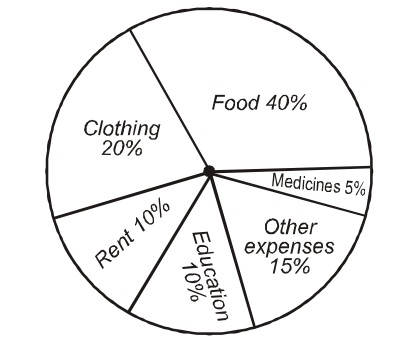Home » Data Interpretation » Pie chart » Question

#### Data Interpretation

Direction: The pie-chart given below shows the household expenditure of a family on different items. Study the chart carefully and answer the questions that follows.1. What is the angle subtended on the center by the segment representing other expenses?
1. 60°
2. 54°
3. 36°
4. 15°
##### Correct Option: B

As per graph,
other expenses = 15 % of total expenditure.
As we know that the angle made on center in a circle is 360°
Since 100% made an angle 360° in a circle.
therefore 1% made an angle 360° / 100 in a circle.
therefore 15% made an angle 15 x 360° / 100 in a circle.
Central angle for 15% =15 x 36° / 10
Central angle for 15% =3 x 36° / 2
Central angle for 15% =3 x 18° = 54°